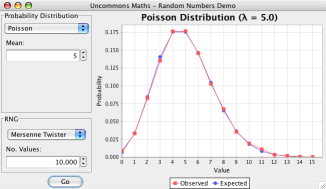# Uncommons Maths

## Random number generators, probability distributions, combinatorics and statistics for Java

Uncommons Maths is Open Source software, free to download and use subject to the terms of the Apache Software Licence, Version 2.0.

## Random Numbers

A Java Programmer's Guide to Random Numbers: Part 1: Beyond java.util.Random · Part 2: Not just coins and dice · Part 3: SeedingThe Uncommons Maths library provides five easy-to-use, statistically sound, high-performance pseudorandom number generators (RNGs). They are:

MersenneTwisterRNG
A Java port of the fast and reliable Mersenne Twister RNG originally developed by Makoto Matsumoto and Takuji Nishimura. It is faster than `java.util.Random`, does not have the same statistical flaws as that RNG and also has a long period (219937).
The Mersenne Twister is an excellent general purpose RNG.
XORShiftRNG
A Java implementation of the very fast PRNG described by George Marsaglia. It has a period of about 2160, which although much shorter than the Mersenne Twister's, is still significantly longer than that of `java.util.Random`.
This is the RNG to use when performance is the primary concern. It can be up to twice as fast as the Mersenne Twister.
CMWC4096RNG
A Java implementation of a Complementary-Multiply-With-Carry (CMWC) RNG as described by George Marsaglia. It has an extremely long period (2131104) and performance comparable to the Mersenne Twister (though the Mersenne Twister has the advantage of only requiring 16 bytes of seed data rather than the 16 kilobytes required by the CMWC RNG).
AESCounterRNG
This is a cryptographically-strong1 non-linear RNG that is around 10x faster than `java.security.SecureRandom`. Reverse-engineering the generator state from observations of its output would involve cracking the AES block cipher.
CellularAutomatonRNG
A Java port of Tony Pasqualoni's fast Cellular Automaton RNG. It uses a 256-cell automaton to generate random values.
1. The algorithm is not the only security consideration for RNGs. The source, secrecy and integrity of the seed data is also vital. For highly sensitive applications, consider using something like Fortuna.

### Probability Distributions

Using the included probability distribution wrappers, these RNGs (and the standard JDK ones) can be used to generate values from Uniform, Normal, Binomial, Poisson and Exponential distributions.

Launch the Uncommons Maths demo application (requires Java WebStart) to experiment with the different distributions.

### Seed Strategies

Good RNGs need good seed data. Uncommons Maths provides pluggable seeding strategies, including ones to read random data from `/dev/random` (where available) and from random.org.

## Combinatorics

Uncommons Maths also includes generics-enabled combination and permutation generators. These are based on Java classes originally written by Michael Gilleland.

## Statistics

Uncommons Maths provides a statistical data set class that can calculate a variety of descriptive statistics (variance, median, standard deviation, arithmetic and geometric means, etc.) for a set of values.

## Rational Arithmetic

Uncommons Maths also includes a `Rational` number type that allows exact fractional arithmetic without loss of precision.

## Binary

Classes for manipulating binary values.